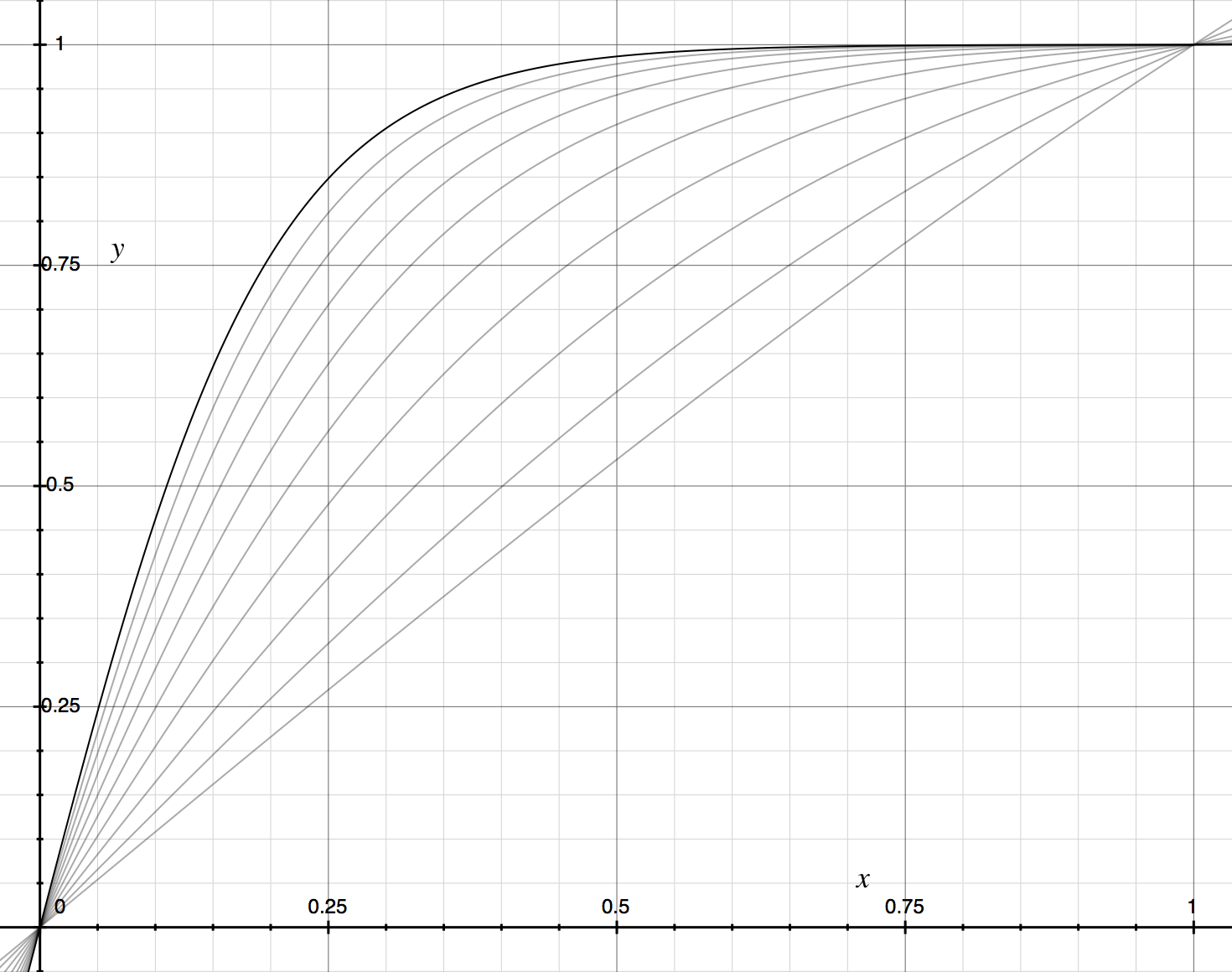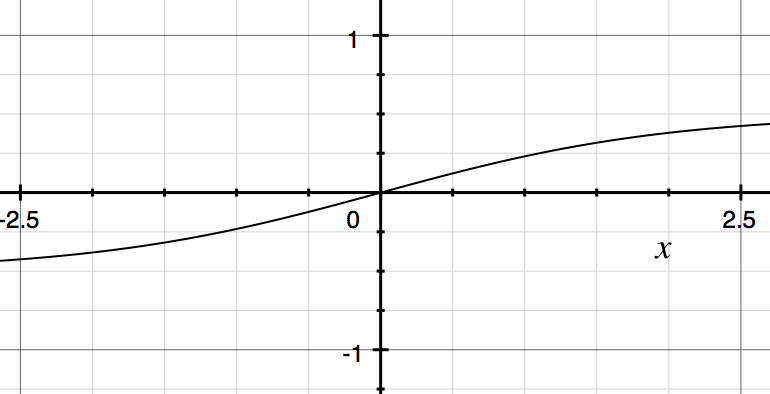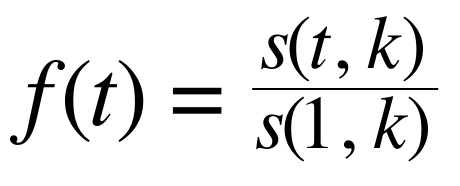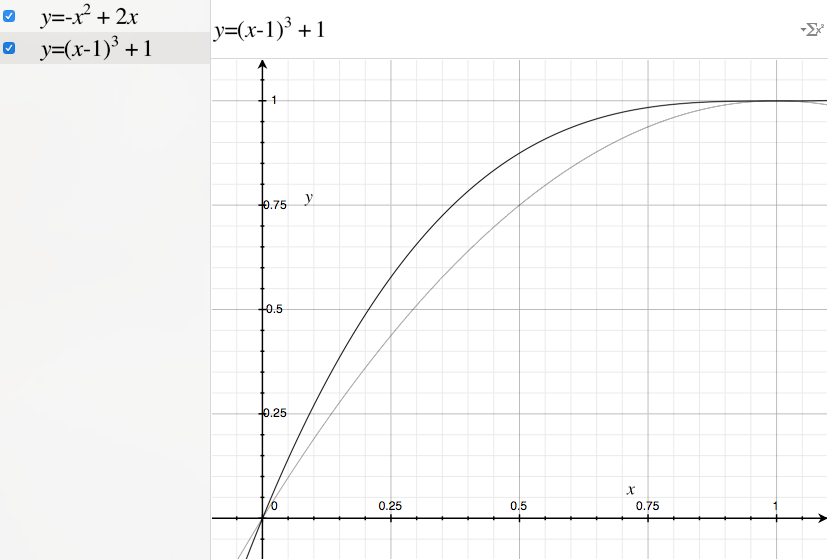# Ease Out: The Half Sigmoid

### Animate your web applications with a customizable ease-out.Ease-out: 9 different versions plotted.

While working on the Explore page and some other features in Eyewire, Alex Norton, Chris, and I developed some techniques to make our videos and animations smoother. I wrote a bunch of our work on easing curves up on Medium, and in this series, I’ll be cross posting to Eyewire so that you guys can see what we’re working on under the hood. Feedback is welcome! – Will

Ease-out animations are great for creating animations that feel like they respond instantly but still cruise to a gentle halt. Most easings specify one of a small number of easing curves: easeOutQuad, easeOutSine, easeOutCubic, etc. However, the sharpness of that curve is not configurable. Here I show how to create a configurable ease-out function that will work for animating any property you desire.

### How to Use It

The easing function takes a single parameter k, that specifies the steepness of the curve. The larger k is, the steeper the curve, and the higher the velocity in the middle section relative to the beginning and ending.

The easeOutFactory will produce a function that takes a parameter t that represents the percentage of the animation completed as a number between 0 and 1. It’s important to note that this system only produces numbers that you can use within an animation framework (that you might have to build). An example framework is included in the jsFiddle.

Here is an example of the usage:

```// LISTING 1 (Javascript)
// easeOutFactory produces a function representing an ease-out.
var easing = easeOutFactory(4); // choose k = 4, see Fig. 2
easing(0.00); // 0
easing(0.10); // 0.2047403251776069
easing(0.25); // 0.4793609299265755
easing(0.50); // 0.7900128291929867
easing(0.75); // 0.9389236079466523
easing(0.90); // 0.9821358147987899
easing(1.00); // 1```

You can design your easing curve visually using this OS X Grapher file. Modify k to adjust the steepness.

### The Theory

This section is not required for use. Read on to understand the techniques underlying the code so that you will be able to develop your own easing curves.

The basic idea is to take the ease-in-out curve developed in Ease In, Out: The Sigmoid Factory and use the right half of it to get our adjustable ease-out.

At the conclusion of The Sigmoid Factory, we had this equation developed to describe a sigmoid centered at the origin:Equation 1: An instrumented sigmoid centered at x = 0. α is our knob to tune the steepness, and t is our animation progression from 0 to 1.Figure 1: The centered sigmoid function from Eqn. 2.

For our animations, we constrain the useful region of the function to be between 0 and 1 on the time (x)and distance (y) axes. Notice how the plotted function is substantially less than 1 at x = 1 in Fig. 1. We can apply the same scaling trick as we used in The Sigmoid Factory to bump it up to 1. That is to say, we normalize the function by its value at x = 1.

Because we’re not shifting the function around, just scaling it, the math works out much more simply than before.Equation 2: The ease out equation. s(t, k) is as specified in Eqn. 1. t is time, k is a steepness constant.Figure 2: The Ease Out Function from Eqn. 2 plotted as k = 4.

You can find the grapher file here.

I’ll leave Ease-In as an exercise to the reader. Hint: Think about how to flip things around. 😘

#### Versus traditional ease-outs

There is a slight deficiency with this method versus easeOutQuad and easeOutCubic.Figure 3: easeOutCubic (black) and easeOutQuad (gray)

You’ll notice that at x = 1, both of these eases have zero vertical velocity. That is to say, their dy/dx at 1 is 0. The adjustable sigmoid ease described here gets quite close to zero starting around k = 6 or 7. If you’re using a kbelow this range, it might look a bit nicer to use one of the traditional eases.

```Table 1: Approximate Corresponding Eases
EASING           EQN            K APPROX.
easeOutSine      sin(2πt/4)     3.25
easeOutQuad      -t^2 + 2t      3.50
easeOutCubic     (t-1)^3 + 1    5.50
easeOutQuint     (t-1)^5 + 1    8.00```

If you enjoyed this article, you might also like The Sigmoid Factory, The Bounce Factory, and The Spring Factory.

### References

1. Ease In, Out: The Sigmoid Factory — Where the theory for the ease-in-out curve used in this article was developed.
2. http://easings.net/ — A collection of many commonly used easing curves.
3. Ease Out Grapher File— A Grapher file I developed to design my ease out functions.

This article was cross posted from “Ease Out: The Half Sigmoid” by Will Silversmith.